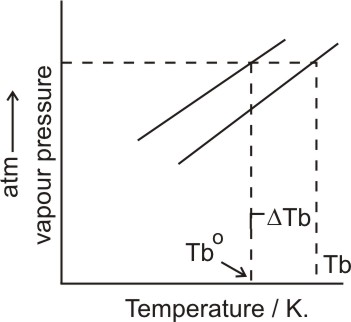# (a) Draw the graph between vapour pressure and temperature and explain the elevation in boiling point of a solvent in solution.(b) Determine the osmotic pressure of a solution prepared by dissolving 25mg of  in 2 litres of water at  assuming it to be completely dissiciated.(Atomic masses K = 39u, S = 32u, O= 16u)

(a) On addition of a solute to a solvent, boiling point of the solution increases. Non-volatile solute when added to a solvent, lowers the vapour pressure and hence boiling point increases.= elevation of boiling point

= vapour pressure of pure solvent

=  vapour pressure of the solution

Addition of non-volatile solute in a solvent, decreases the number of volatile particles on the surface of the solution. Hence, the particles that vapourises out from the surface decreases and hence decreases the vapour pressure of the solution. The elevation of boiling point is given by the formula.

(b) Weight of the solute

Volume of the elevation (V) = 2L

Molar mass of solute  =174g/mol

Gas constant (R) = 0.0821 LatmK-1

Temperature (T) =  = 25+273 = 298K

Osmotic pressure = ?

## Related Chapters

### Preparation Products

##### Knockout NEET Sept 2020

An exhaustive E-learning program for the complete preparation of NEET..

₹ 15999/- ₹ 6999/-
##### Rank Booster NEET 2020

This course will help student to be better prepared and study in the right direction for NEET..

₹ 9999/- ₹ 4999/-
##### Knockout JEE Main Sept 2020

An exhaustive E-learning program for the complete preparation of JEE Main..

₹ 12999/- ₹ 6999/-
##### Test Series NEET Sept 2020

Take chapter-wise, subject-wise and Complete syllabus mock tests and get in depth analysis of your test..

₹ 4999/- ₹ 2999/-